# Pandas Groupby Tutorial

Hope if you are reading this post then you know what is groupby in SQL and how it is being used to aggregate the data of the rows with the same value in one or more column. I was recently working on the Pandas Groupby and found there are lot of useful features which can be used to explore the data and this triggered me to write this post so that anyone with a SQL groupby knowledge can learn the Pandas group by within no time. In this blog I am going to take a dataset and show how we can perform groupby on this data and explore the data further.

We are going to use the seaborn exercise data for this tutorial. The data represents the type of diet and its corresponding pulse rate measured for the time in mins. You can load this data with a simple searborn command and then after some cleanup the data is ready to be used

``````import seaborn as sns
import pandas as pd

exercise.drop('Unnamed: 0',inplace=True,axis='columns')
exercise['time']=exercise['time'].str.replace(' min','')
exercise['time']= pd.to_numeric(exercise['time'])
exercise.rename(columns={'time':'time_mins'},inplace=True)
``````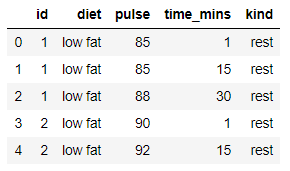## Pandas Groupby Count

As a first step everyone would be interested to group the data on single or multiple column and count the number of rows within each group. So you can get the count using size or count function. if you are using the count() function then it will return a dataframe. Here we are interested to group on the id and Kind(resting,walking,sleeping etc.) when the pulse rate is measured. You can see for the id: 1 and kind resting the data has 3 rows in it and for walking and running there are no rows available in the data.

``````grouped=exercise.groupby(['id','kind'],axis=0)
grouped.count()
``````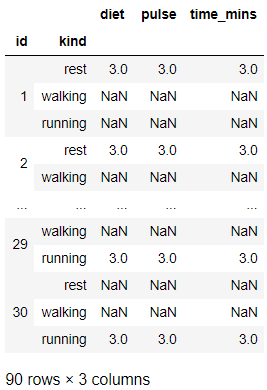## Basic Aggreggation

Now lets look at the simple aggregations functions that can be applied on the columns for this data. So if you have seen this data then the first thing you would be interested to know is what is the mean or average pulse rate across each of the diet under each id. Here we will first group by id and diet and then use the mean function to get a multi-index dataframe of the groups with the mean values for the column pulse and time_mins. We can easily find it out from this data that diet with low fat gives less pulse rate than the diet with no fat. Wow so we cleared the misconception with this data that eating fat rich food is not good for health.

``````exercise.groupby(['diet']).mean()
``````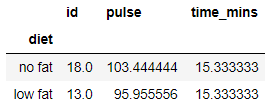I hope at this point of time you would also be interested to see what is the average pulse for each of the kind. so lets find it out. Looks like resting has lowest mean pulse rate and running has the highest which was expected.

``````exercise.groupby(['kind']).mean()
``````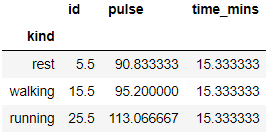There are other aggregating functions like sum, min, max, std,var etc. We will look into some of these functions later in the post. You can check these other functions

### Aggregating functions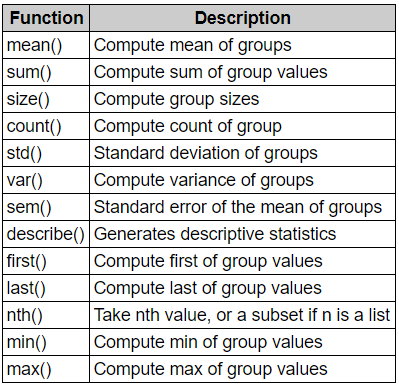The result of the aggregation will have the group names as the new index along the grouped axis. In the case of multiple keys, the result is a Multi-Index by default, though this can be changed by using the as_index option. You can set the as_index parameter as False

``````exercise.groupby(['id','diet'],as_index=False).agg(sum).head()
``````

or

You can also do a reset_index

``````exercise.groupby(['id','diet']).sum().head().reset_index()
``````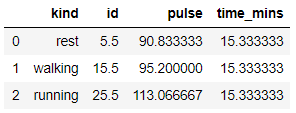### Describe

if you want to generate a descriptive statistics that summarize the count, mean, std deviation, percentile and max values of a datasets distribution then simply use the describe function on the groupby object

``````grouped=exercise.groupby(['id','diet'])
``````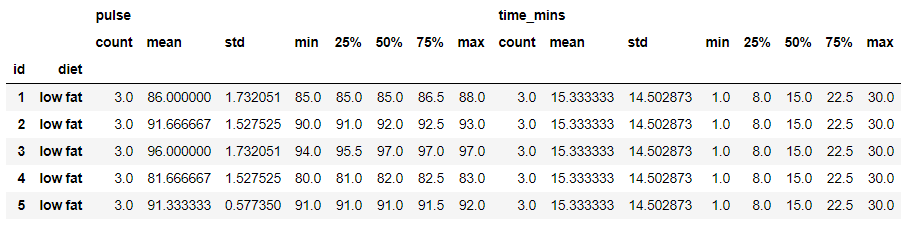## Pandas Groupby Multiple Functions

With a grouped series or a column of the group you can also use a list of aggregate function or a dict of functions to do aggregation with and the result would be a hierarchical index dataframe

``````exercise.groupby(['id','diet'])['pulse'].agg(['max','mean','min']).head()
``````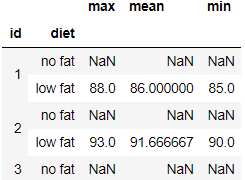Similarly on a groupby object you can pass list of functions and it will give the aggregated results for all the columns in the group

``````exercise.groupby(['id','diet']).agg(['max','mean','min']).head()
``````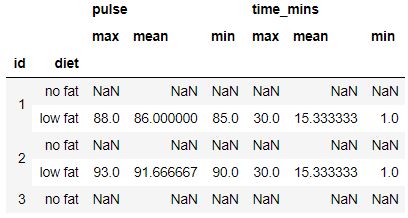## Lambda function for Aggreggation

You can also use a lambda function for aggregation with the groupby object. So here I am looking for a lambda function on the groupby which will give me the diff of max and min value in each group for both the columns pulse and time. The output will be a multi-index dataframe object and also renaming the column to diff

``````grouped = exercise.groupby(['id','diet']).agg([lambda x: x.max() - x.min()]).rename(columns={'<lambda>': 'diff'})
``````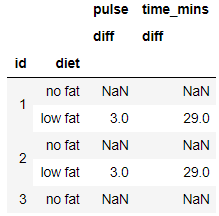## Pandas groupby aggregate multiple columns using Named Aggregation

As per the Pandas Documentation,To support column-specific aggregation with control over the output column names, pandas accepts the special syntax in GroupBy.agg(), known as “named aggregation”, where

1. The keywords are the output column names
2. The values are tuples whose first element is the column to select and the second element is the aggregation to apply to that column. Pandas provides the pandas.NamedAgg namedtuple with the fields [‘column’, ‘aggfunc’] to make it clearer what the arguments are. As usual, the aggregation can be a callable or a string alias.

So we can specify for each column what is the aggregation function we want to apply and give a customize name to it.

``````import numpy as np
exercise.groupby(['id','diet']).agg(min_pulse=pd.NamedAgg(column='pulse', aggfunc='min'),
max_time=pd.NamedAgg(column='time_mins', aggfunc='max'),
``````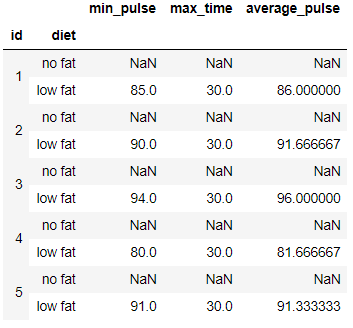## Column Indexing

The groupby object can be indexed by a column and the result will be a Series groupby object. Let’s use series groupby object time_mins and calculate its mean. So we get the total time for each of the kind.

``````exercise.groupby('kind')['time_mins'].mean()
``````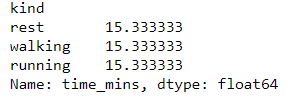``````exercise.groupby('kind')['pulse'].mean()
``````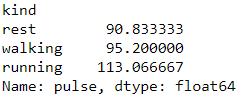## Pandas groupby get_group

Another useful method to select a group from the groupby object so from the groupby object we want to get kind - walking and it gives a dataframe with all rows of walking group. Basically it gets you all the rows of the group you are seeking for

``````grouped=exercise.groupby('kind')
``````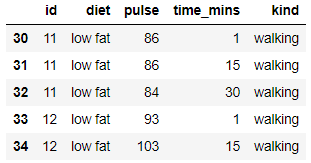for an object grouped on multiple columns:

``````grouped=exercise.groupby(['kind','diet'])
``````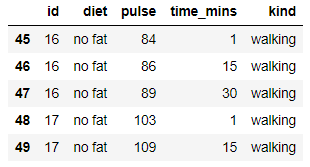## Iterating groupby

if you want to iterate through each group for some manual operation then you can use something like this and it will return either a series or dataframe

``````for name, group in grouped:
print(name)
print(group)
``````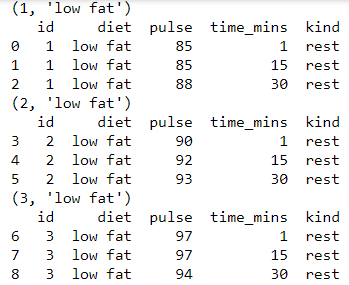## Pandas SQL groupby Having

you can query the multi-index dataframe using query function or use filter. Read this blog on how to use filters on groupby object

``````grouped=exercise.groupby(['id','diet']).agg('count').head()
# Same as SQL having
grouped.query('pulse > 2')
``````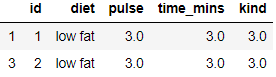## Groupby Cumulative Sum

So you want to do a cumulative sum of all the pulse and time_mins for each group, which means to add up those column values for each group

``````exercise.groupby(['id','diet']).agg(sum).groupby('diet').cumsum()
``````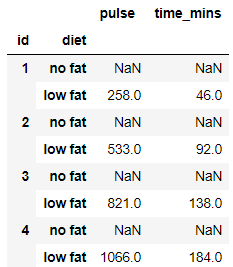## Filtering Multi-index Columns

There is a small work around for filtering the multi-index grouped dataframe. Suppose you want to get all the rows where pulse max,min difference is greater than 10 and time_mins max value is greater than or equal to 30

``````grouped[(grouped[('pulse','diff')]>10) & (grouped[('time_mins','max')]>=30)]
``````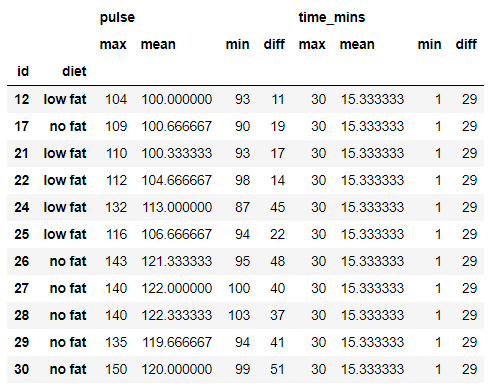## Transform and Filter

Using transform you can create a new column with the aggregated data and get your original dataframe back. Whereas filter can be used like having in SQL. I have a detailed blog which talks about how to use Transform and Filter with groupby. Please check this link.

## Groupby Apply Function

We can also use apply and pass a function to each group in the groupby object. Say you want to half the pulse-rate in each group, so we can group it by id first and then use apply and pass our customized function so that it will return a dataframe with all the rows of the group and their halved pulse rate.

``````def divide_by_half(x):
# x is a DataFrame of group values
x['pulse']=x['pulse']/2
return x

exercise.groupby('id').apply(norm_by_data2)
``````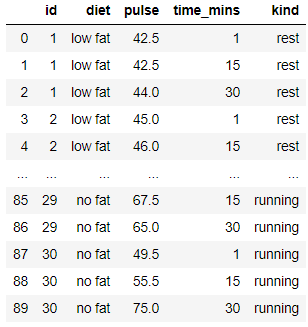## Pandas groupby aggregate to list

Many a times we have seen instead of applying aggregation function we want the values of each group to be bind in a list. So if you want to list of all the time_mins in each group by id and diet then here is how you can do it

``````exercise.groupby(['id','diet'])['time_mins'].apply(list)
``````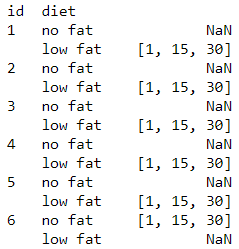## Conditional Group by count

This is an interesting one. Suppose you want to group the data on id and diet and want to count all the pulse which is equal to 85

``````exercise.groupby(['id','diet'])['pulse'].apply(lambda x: x[x == 85].count())
``````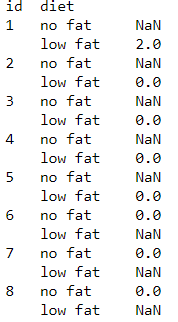This post was a very detailed introduction to pandas group by and all the features and functions that can be used along with it. As a next step you can run these codes and play around with other aggregation functions and get into the details of the code and can get many more interesting results. It’s not possible to cover all the scenarios and use cases around the groupby in one blog post. I will try to cover other features and use cases in my upcoming blogs. Let me know if you find this blog useful or do you have any suggestions in the comments sections below.

Tags:

Categories:

Updated: